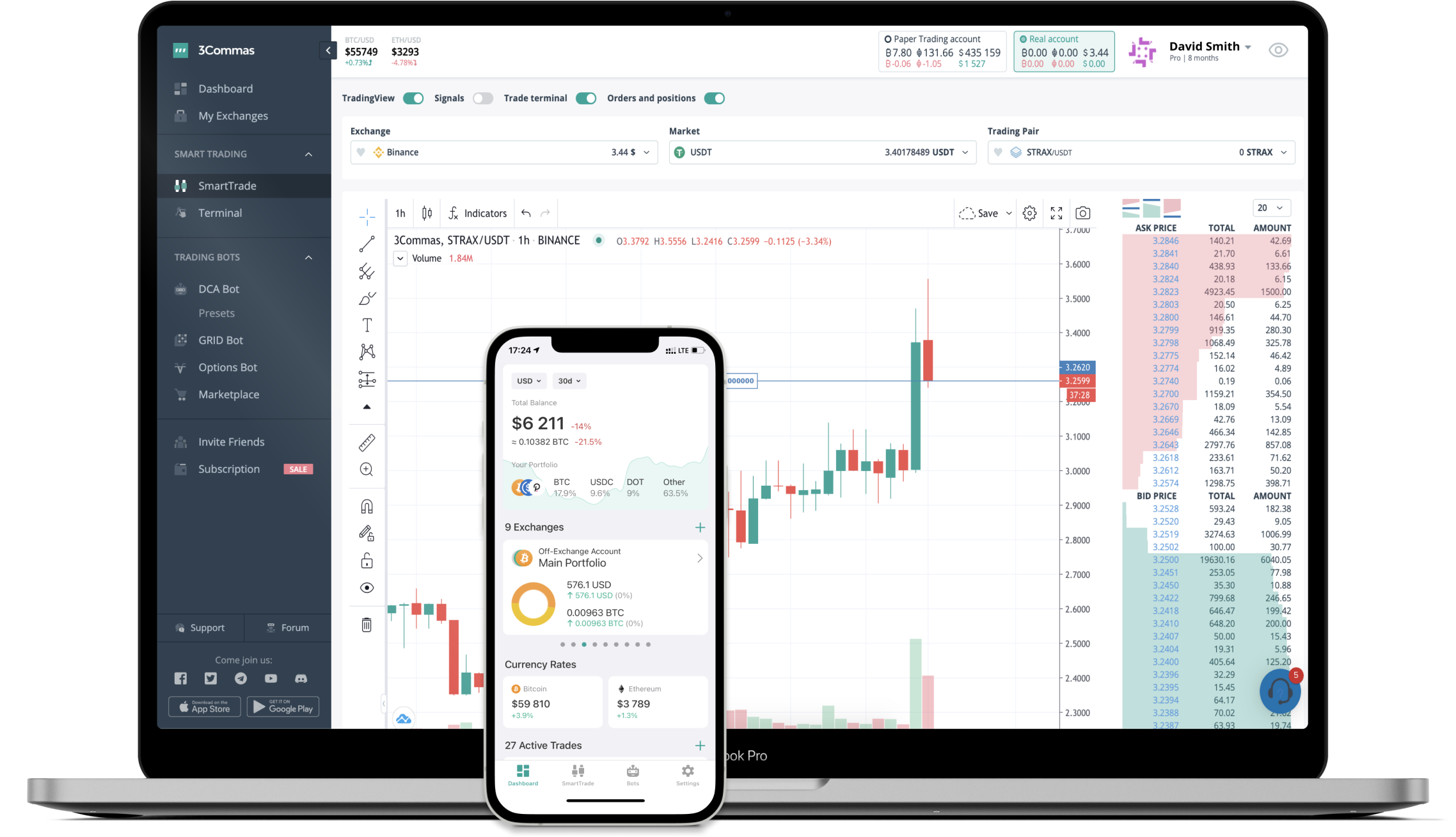# DELTA to RUB Converter

Delta Financial
PriceRUB 87.8+0.65%
VolumeRUB 7,308
How it work

The 3Commas currency calculator allows you to convert a currency from Delta Financial (DELTA) to Russian Ruble (RUB) in just a few clicks at live exchange rates.

Simply enter the amount of Delta Financial you wish to convert to RUB and the conversion amount automatically populates. You can also use our Prices Calculator Table to calculate how much your currency is worth in other denominations, i.e. .1 DELTA, .5 DELTA, 1 DELTA, 5 DELTA, or even 10 DELTA.

## DELTA to RUB Exchange Calculator

The chart displays Delta Financial price changes in RUB.

The ultimate tools for crypto traders to maximize trading profits while minimizing risk and loss.## Latest DELTA to RUB price calculator

Delta Financial market price is updated every three minutes and is automatically displayed in RUB. Below are the most popular denominations to convert to RUB.

Delta Financial
Russian Ruble
0.88
RUB
8.78
RUB
87.80
RUB
175.60
RUB
263.40
RUB
439.00
RUB
878.00
RUB
2195.00
RUB
4390.00
RUB
8780.00
RUB
21950.00
RUB
43900.00
RUB
87800.00
RUB
219500.00
RUB
Russian Ruble
Delta Financial
0.00011390
DELTA
0.00113895
DELTA
0.01138952
DELTA
0.02277904
DELTA
0.03416856
DELTA
0.05694761
DELTA
0.11389522
DELTA
0.28473804
DELTA
0.56947608
DELTA
1.13895216
DELTA
2.84738041
DELTA
5.69476082
DELTA
11.38952164
DELTA
28.47380410
DELTA

## FAQ

### How much is 1 Delta Financial in Russian Ruble?

Delta Financial price in RUB is constantly changing.

At this moment, 1 Delta Financial equals 87.8 RUB

### How do I calculate the conversion from DELTA to RUB?

The 3Commas Delta Financial Calculator allows you to easily calculate the conversion price of DELTA to RUB by simply entering the amount of Delta Financial in the corresponding field and will automatically convert the value in Russian Ruble (RUB).

You can also use our Delta Financial price table above to check the latest Delta Financial price in major fiat and crypto currencies.

### How can I convert Delta Financial to RUB?

The most common way of converting DELTA to RUB is by using a Crypto Exchange or a P2P (person-to-person) exchange platform like LocalBitcoins, etc.